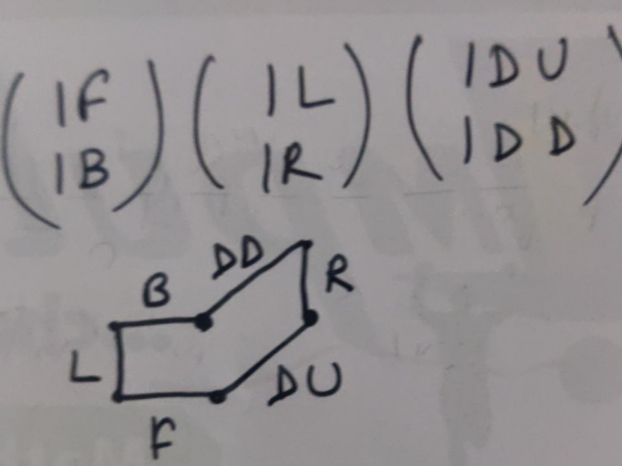# Doubt from permutations and combinations

Question 27

My logic was that after every step king has 8 options and that the total number of different paths would be equal to the number of ways he could come back

\bullet\; One Direction:

\displaystyle\bigg(3\text{F},3\text{B}\bigg)=\binom{4}{1}\cdot \frac{6!}{3!\cdot 3!}=80.

\bullet\; Two Direction:

\displaystyle\bigg(2\text{F},2\text{B}\bigg)+\bigg(1\text{R},1\text{L}\bigg)=\frac{6!}{2!\cdot 2!}\cdot \binom{4}{2}\cdot 2=2160.

\bullet\; Three Direction:

\displaystyle\bigg(1\text{F},1\text{B}\bigg)+\bigg(1\text{R},1\text{L}\bigg)+\bigg(1\text{D},1\text{DBa}\bigg)=6!\cdot \binom{4}{3}=2880.

So we get total ways

\color{brown}=80+2160+2880=5120.Note: Solution is not completely mine. I have Taken some help from my friend.

2 Likes

In between Brackets, There is no plus sign.

Done by mistake.

Diagram for 2 nd and 3 rd cases.

1 Like

@Jagdish_Singh. Sir In second and third steps what are the 4C2 and 4C3 respectively for ?
I understand that 6!/(2!*2! ) Is essentially to arrange the steps

@Jagdish_Singh sir is the 4c1 for selecting direction of movement?